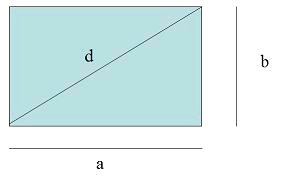# The rectangleThe area of the rectangle is: $$A=a \cdot b$$$Its perimeter is: $$P=a+a+b+b=2a+2b=2(a+b)$$$

And, applying the Pythagorean theorem, its diagonal is: $$d=\sqrt{a^2+b^2}$$$Calculate the diagonal and the area of a rectangle of $$5 \ cm \times 10 \ cm$$. • Diagonal $$d^2=(5 \ cm)^2+(10 \ cm )^2 \\ d = \sqrt{25+100} \ cm \\ d= 5\sqrt{5} \ cm$$$

• Area $$A= 5 \ cm \cdot 10 \ cm = 50 \ cm^2$$\$

And so, the area of the rectangle is: $$50 \ cm^2$$.